## Chapter 4Some remarks on GLn(Fq)

In this chapter we state some results on the representation theory of GLn(Fq), without proofs, with the intention of motivating further reading. The construction of representations of GLn(Fq) follows that same principles as in the case of GL2(Fq). Parabolic induction (of which the constructions in Chapter 2 are examples) is used to construct a large number of irreducible representations of GLn(Fq) from representations of GLm(Fq), when m < n. The parameterisation of such representations is, in some sense, related to the representation theory of symmetric groups. The remaining representations are called cuspidal and are parameterised by the Galois orbits of primitive characters of Fqn*. The irreducible representations come in families, which reflect the parametrisation of conjugacy classes on GLn(Fq).

### 4.1. Parabolic Induction

The process of parabolic induction is best thought of in terms of a graded associative algebra. Let Rn denote the free abelian group generated by the set of isomorphism classes of irreducible representations of GLn(Fq). Set R = n=1Rn. Let Pn,n denote the subgroup of GLn+n(Fq) consisting of matrices with block form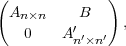where A and Aare in GLn(Fq) and GLn(Fq) respectively, and B is an arbitrary matrix of the appropriate size. Given representations (π,V ) and (π,V ) of GLn(Fq) and GLn(Fq) respectively, let π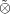πbe the representation of Pn,n on V V defined by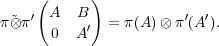Define π πto be the representation of (ππ)GLn+n(Fq) of GLn+n(Fq) [Gre55, p.403]. This binary operation Rn × RnRn+n can be extended linearly to R. Green shows that this is a commutative and associative product on R.

### 4.2. Cuspidal representations

The cuspidal representations of GLn(Fq) are those which are disjoint from all representations of the form π′∘ π′′, where πand π′′ are irreducible representations of GLn(Fq) and GLn′′(Fq), where n = n+ n′′ and nand n′′ are both positive.

Together with the ‘’ operation, cuspidal representations generate all of R.

The cuspidal representations of GLn(Fq) have a nice parametrisation. A character ω of Fqn* is called primitive if there does not exists any dn such that ω = N χ for any character χ of Fqd*. Here N denotes the norm map Fqn Fqd (see Section B.3). The Galois group of Fqn over Fq acts on the set of primitive characters of Fqn: ωg(x) = ω(gx) for an element g of the Galois group, for each x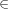Fqn.

Theorem 4.1. There is a canonical bijective correspondence between the set of Galois orbits of primitive characters of Fqn* and isomorphism classes irreducible cuspidal representations of GLn(Fq).

It should be noted that the number such orbits is the same as the number of irreducible monic polynomials of degree n with coefficients in Fq. These correspond precisely to the conjugacy classes of matrices in GLn(Fq) with irreducible characteristic polynomial. Moreover, this correspondence has a nice manifestation in terms of character values.

Theorem 4.2. Let f(t) is an irreducible monic polynomial of degree n with coefficients in Fq with roots z1,,zn in Fqn, and let ω be a primitive character of Fqn*. Let πω denote the irreducible cuspidal representation of GLn(Fq) corresponding to the Galois orbit of ω. Then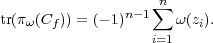The primary decomposition for matrices (Corollary A.13) has an analogy for representations of GLn(Fq). Fix an irreducible cuspidal representation π of some GLn(Fq). Say that a representation ρ of GLm(Fq) is π-primary if it is a subrepresentation of some polynomial expression of π in R. If ρ1,n are irreducible primary representations, with ρi begin πi-primary, where π1,n are pairwise non-isomorphic cuspidal representations, then ρ1ρn is irreducible.

Green shows that the irreducible π-primary representations are parameterised by partitions. It is no coincidence that the irreducible representations of symmetric groups are also parameterised by partitions. An elegant approach to understanding these relationships is by putting additional structure on R, namely that of a positive self adjoint Hopf algebra. Very general results about the structure of such algebras are interpreted in terms of the representation theory of general linear groups over finite fields by Zelevinsky in [Zel81].